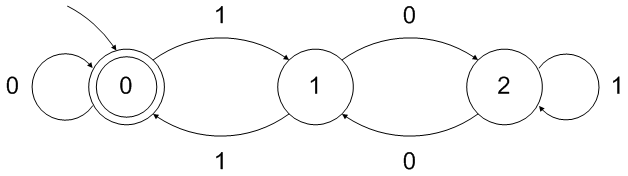# GATE | GATE CS 2021 | Set 2 | Question 57

• Last Updated : 23 May, 2021

Which of the following regular expressions represent(s) the set of all binary numbers that are divisible by three? Assume that the string ϵ is divisible by three.
(A) (0+1(01*0)*1)*
(B) (0+11+10(1+00)*01)*
(C) (0*(1(01*0)*1)*)*
(D) (0+11+11(1+00)*00)*

Explanation: Binary numbers divisible by 3 fall into 3 categories:

Numbers with two consecutive 1’s or two 1’s separated by an even number of 0’s. Effectively every pair “cancels” itself out.
(ex. 11, 110, 1100,1001,10010, 1111)

(decimal: 3, 6, 12, 9, 18, 15)

Numbers with three 1’s each separated by an odd number of 0’s. These triplets also “cancel” themselves out.
(ex. 10101, 101010, 1010001, 1000101)

(decimal: 21, 42, 81, 69)

Some combination of the first two rules (including inside one another)
(ex. 1010111, 1110101, 1011100110001)

(decimal: 87, 117, 5937)

So a regular expression that takes into account these three rules is simply:

0*(1(00)*10*|10(00)*1(00)*(11)*0(00)*10*)*0*Quiz of this Question

My Personal Notes arrow_drop_up
Recommended Articles
Page :